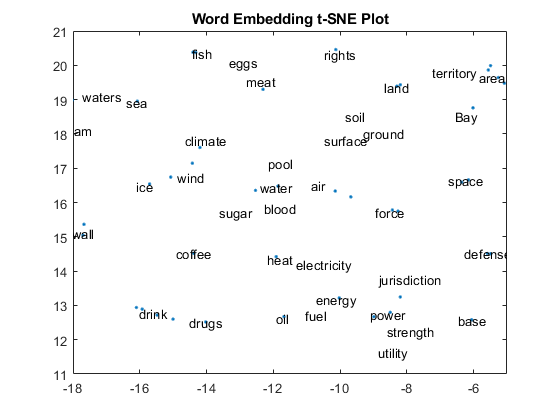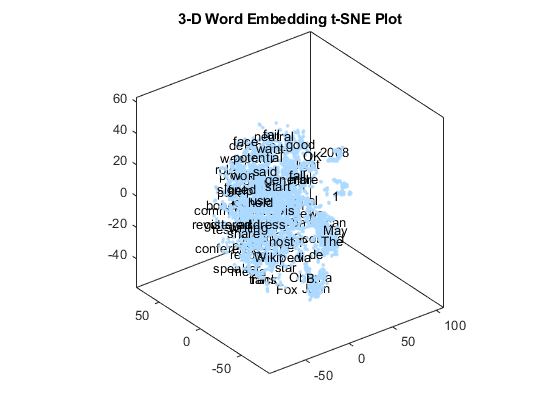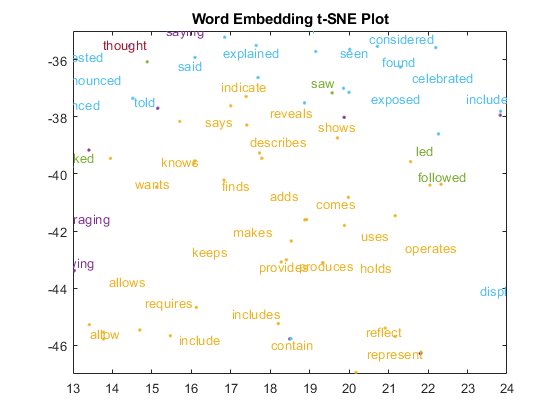Documentation

## Visualize Word Embeddings Using Text Scatter Plots

This example shows how to visualize word embeddings using 2-D and 3-D t-SNE and text scatter plots.

Word embeddings map words in a vocabulary to real vectors. The vectors attempt to capture the semantics of the words, so that similar words have similar vectors. Some embeddings also capture relationships between words like "Italy is to France as Rome is to Paris". In vector form, this relationship is $\mathit{Italy}-\mathit{Rome}+\mathit{Paris}=\mathit{France}$.

To reproduce the results in this example, set `rng` to `'default'`.

`rng('default')`

Load a pretrained word embedding using `fastTextWordEmbedding`. This function requires Text Analytics Toolbox™ Model for fastText English 16 Billion Token Word Embedding support package. If this support package is not installed, then the function provides a download link.

`emb = fastTextWordEmbedding`
```emb = wordEmbedding with properties: Dimension: 300 Vocabulary: [1×999994 string] ```

Explore the word embedding using `word2vec` and `vec2word`. Convert the words Italy, Rome, and Paris to vectors using `word2vec`.

```italy = word2vec(emb,"Italy"); rome = word2vec(emb,"Rome"); paris = word2vec(emb,"Paris");```

Compute the vector given by `italy - rome + paris`. This vector encapsulates the semantic meaning of the word Italy, without the semantics of the word Rome, and also includes the semantics of the word Paris.

`vec = italy - rome + paris`
```vec = 1×300 single row vector 0.1606 -0.0690 0.1183 -0.0349 0.0672 0.0907 -0.1820 -0.0080 0.0320 -0.0936 -0.0329 -0.1548 0.1737 -0.0937 -0.1619 0.0777 -0.0843 0.0066 0.0600 -0.2059 -0.0268 0.1350 -0.0900 0.0314 0.0686 -0.0338 0.1841 0.1708 0.0276 0.0719 -0.1667 0.0231 0.0265 -0.1773 -0.1135 0.1018 -0.2339 0.1008 0.1057 -0.1118 0.2891 -0.0358 0.0911 -0.0958 -0.0184 0.0740 -0.1081 0.0826 0.0463 0.0043 ```

Find the closest words in the embedding to `vec` using `vec2word`.

`word = vec2word(emb,vec)`
```word = "France" ```

### Create 2-D Text Scatter Plot

Visualize the word embedding by creating a 2-D text scatter plot using `tsne` and `textscatter`.

Convert the first 500 words to vectors using `word2vec`. `V` is a matrix of word vectors of length 300.

```words = emb.Vocabulary(1:5000); V = word2vec(emb,words); size(V)```
```ans = 1×2 5000 300 ```

Embed the word vectors in two-dimensional space using `tsne`. This function may take a few minutes to run. If you want to display the convergence information, then set the `'Verbose'` name-value pair to 1.

`XY = tsne(V);`

Plot the words at the coordinates specified by `XY` in a 2-D text scatter plot. For readability, `textscatter`, by default, does not display all of the input words and displays markers instead.

```figure textscatter(XY,words) title("Word Embedding t-SNE Plot")```Zoom in on a section of the plot.

```xlim([-18 -5]) ylim([11 21])```### Create 3-D Text Scatter Plot

Visualize the word embedding by creating a 3-D text scatter plot using `tsne` and `textscatter`.

Convert the first 5000 words to vectors using `word2vec`. `V` is a matrix of word vectors of length 300.

```words = emb.Vocabulary(1:5000); V = word2vec(emb,words); size(V)```
```ans = 1×2 5000 300 ```

Embed the word vectors in a three-dimensional space using `tsne` by specifying the number of dimensions to be three. This function may take a few minutes to run. If you want to display the convergence information, then you can set the `'Verbose'` name-value pair to 1.

`XYZ = tsne(V,'NumDimensions',3);`

Plot the words at the coordinates specified by XYZ in a 3-D text scatter plot.

```figure ts = textscatter3(XYZ,words); title("3-D Word Embedding t-SNE Plot")```Zoom in on a section of the plot.

```xlim([12.04 19.48]) ylim([-2.66 3.40]) zlim([10.03 14.53])```### Perform Cluster Analysis

Convert the first 5000 words to vectors using `word2vec`. `V` is a matrix of word vectors of length 300.

```words = emb.Vocabulary(1:5000); V = word2vec(emb,words); size(V)```
```ans = 1×2 5000 300 ```

Discover 25 clusters using `kmeans`.

`cidx = kmeans(V,25,'dist','sqeuclidean');`

Visualize the clusters in a text scatter plot using the 2-D t-SNE data coordinates calculated earlier.

```figure textscatter(XY,words,'ColorData',categorical(cidx)); title("Word Embedding t-SNE Plot")```Zoom in on a section of the plot.

```xlim([13 24]) ylim([-47 -35])```